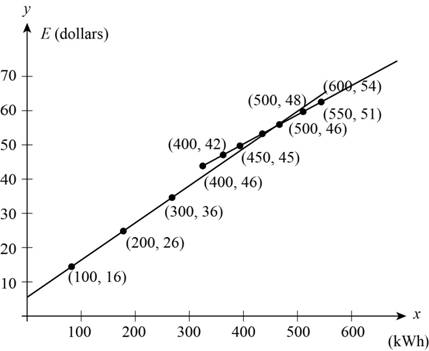# To express: The cost E as a piecewise defined function.### Precalculus: Mathematics for Calcu...

6th Edition
Stewart + 5 others
Publisher: Cengage Learning
ISBN: 9780840068071### Precalculus: Mathematics for Calcu...

6th Edition
Stewart + 5 others
Publisher: Cengage Learning
ISBN: 9780840068071

#### Solutions

Chapter 2.2, Problem 81E

(a)

To determine

## To express: The cost E as a piecewise defined function.

Expert Solution

The piecewise function is E(x)={6+0.1xif(0x300)36+0.06(x300)if(x>300) .

### Explanation of Solution

Given:

Westside charges its electric customers at a rate of \$6.00 per month.

First 300 users charged 10 cent per kilowatt-hour.

Rest user charged 6 cent over 300 kilo watt per hour.

Calculation:

Let users use x kilowatt-hour in one month.

Calculate for (0x300) ,

Price=BasePrice+cents/kWh (1)

Calculate cents/kWh 300 users,

cents/kwh=(10x)=0.1x

Substitute 6 for base price and (10x) for cents/kWh in equation (1),

Price=6+0.1x

Calculate cents/kWh for more than 300 users,

cents/kwh=0.1(300)+0.06(x300)=30+0.06(x300) (2)

Calculate for (x>300) ,

Substitute 6 for base price and equation (2) for cents/kWh in equation (1),

Price=6+30+0.06(x300)=36+0.06(x300)

Thus, the Piecewise defined function x for cost E is,

E(x)={6+0.1xif(0x300)36+0.06(x300)if(x>300) .

(b)

To determine

Expert Solution

### Explanation of Solution

From part (a) the piecewise function is,

E(x)={6+0.1xif(0x300)36+0.06(x300)if(x>300)

Substitute some values in both the functions as in the below table.

 (6+0.1x) (36+0.06(x−300)) x y x y 100 16 400 42 200 26 450 45 300 36 500 48 400 46 550 51 500 56 600 54

From the above table sketch a graph whose values lies from 100 to 60 as in the below Figure 1.Figure (1)

Thus, above graph shows the piecewise function for (0x600) .

### Have a homework question?

Subscribe to bartleby learn! Ask subject matter experts 30 homework questions each month. Plus, you’ll have access to millions of step-by-step textbook answers!